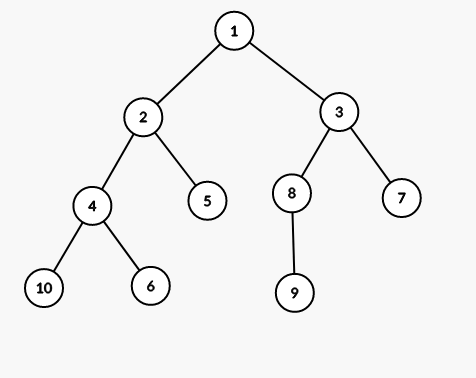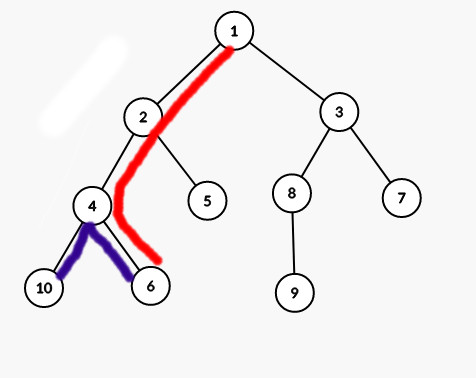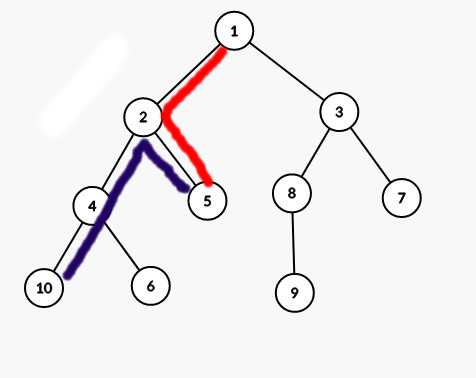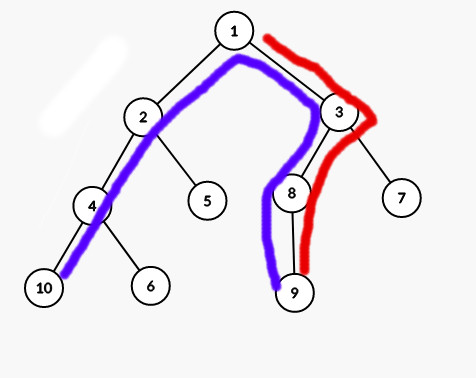# SPTREE - Editorial

Setter:
Tester & Editorialist: Taranpreet Singh

Easy

# PREREQUISITES

Binary Lifting, LCA.

# PROBLEM

Given a tree with N nodes, among with K nodes are marked as special. You are also given a node A. For each node B, determine the maximum value of d(A, X)-d(B, X) where d(a, b) denotes the number of edges on unique path from a to b, and X is any special node. Also, find one X node which maximizes d(A, X)-d(B, X) for each B.

# QUICK EXPLANATION

• Rooting the tree at node A. For node B, it is optimum to choose X such that the depth of LCA(X, B) is maximized.
• It is equivalent to finding the deepest ancestor P of node B such that subtree of P contains atleast 1 special node. Maximum value of d(A, X)-d(B, X) = d(A, P)-d(B, P)

# EXPLANATION

Let’s consider the following tree with A = 1 and consider B = 10, and special nodes are 5, 6 and 9.Following images depict d(A, X) and d(B, X) by red and purple path respectively.
With X = 6With X = 5With X = 9What we are looking for is the maximum value of d(A, X)-d(B, X).

These paths have common path d(L, X) for each X where L is the first common vertex on the path from A to X and path B to X.

If we root the tree on A, the first common vertex L is by definition the Lowest Common Ancestor of B and X.

Now, In order to maximize d(A, X)-d(B, X) = d(A, L)-d(B, L) where L lies on path from A to B, we can see that we need to maximize d(A, L) and minimize d(B, L) which implies we should select L closest to B such that there exists some special node X corresponding to such L.

Restating, for a fixed B, we are looking for the lowest ancestor L of B such that subtree of L contains at least one special node.

If you have managed to follow till here, you have solved the hard part of the problem. All that is left is implementation here.

Let’s assume for each node u, we have computed sp(u) which returns any special descendent in subtree of node u or -1 if there’s no special node in subtree of node u.

Naive way would be to consider each node B one by one and keep moving to its parent till sp(u) \neq -1.

One way to optimize is to notice the fact that if we consider all nodes on path from A to B in the order they appear, some non-prefix of nodes shall have sp(u) \neq -1 and remaining suffix (possibly empty) of nodes in this list shall have sp(u) = -1. We need to find the last node having sp(u) \neq -1.

Hence, we can use binary lifting to find the lowest ansestor of node B.

### Bonus

It is possible to solve this problem in O(N) too.

How?

Run a dfs to find sp(u) for each node u and another dfs passing the deepest node x on path from root to current node having sp(x) \neq -1 shall work. Implementation is left as an exercize.

# TIME COMPLEXITY

The time complexity of binary lifting solution is O(N*log(N)) per test case.

# SOLUTIONS

Setter's Solution O(N)
``````#include <bits/stdc++.h>
using namespace std;
typedef long long ll;
const int N = 3e5+45;

int n,k,a;
int spc[N];
vector <int> g[N];
int has[N];
int dep[N],closest[N],spnode[N];

void prep(int x,int par){
has[x] |= spc[x];

int spn = 0;
for(auto f : g[x]){
if(f == par) continue;
dep[f] = dep[x]+1;
prep(f,x);
has[x] |= has[f];
if(has[f]) spn = spnode[f];
}

if(spc[x]) spnode[x] = x;
else spnode[x] = spn;
}

void dfs(int x,int par){
if(has[x]) closest[x] = x;
else closest[x] = closest[par];
for(auto f : g[x]){
if(f == par) continue;
dfs(f,x);
}
}

void solve(){
cin >> n >> k >> a;
for(int i = 1; i <= n; i++){
g[i].clear();
spc[i] = dep[i] = closest[i] = has[i] = spnode[i] = 0;
}

for(int i = 1; i <= k; i++){
int x;
cin >> x;
spc[x] = 1;
}

for(int i = 1; i < n; i++){
int u,v;
cin >> u >> v;
g[u].push_back(v);
g[v].push_back(u);
}

dep[a] = 0;
prep(a,0);
dfs(a,0);

for(int i = 1; i <= n; i++){
int maxval = 2*dep[closest[i]]-dep[i];
cout << maxval;
if(i < n) cout << " ";
else cout << endl;
}

for(int i = 1; i <= n; i++){
cout << spnode[closest[i]];
if(i < n) cout << " ";
else cout << endl;
}
}

int main(){
ios_base::sync_with_stdio(false);
cin.tie(0);
int t;
cin >> t;
while(t--){
solve();
}
}
``````
Tester's Solution
``````import java.util.*;
import java.io.*;
class SPTREE{
//SOLUTION BEGIN
int B = 18;
void pre() throws Exception{}
void solve(int TC) throws Exception{
int N = ni(), K = ni(), root = ni()-1;
boolean[] special = new boolean[N];
for(int i = 0; i< K; i++)special[ni()-1] = true;
int[] from = new int[N-1], to = new int[N-1];
for(int i = 0; i< N-1; i++){
from[i] = ni()-1;
to[i] = ni()-1;
}
int[][] tree = make(N, N-1, from, to, true);

int[] depth = new int[N];
int[][] par = new int[B][N];
for(int b = 0; b< B; b++)Arrays.fill(par[b], -1);
int[] specialDescendent = new int[N];
Arrays.fill(specialDescendent, -1);
dfs(tree, par, depth, special, specialDescendent, root, -1);

int[] ans = new int[N];
Arrays.fill(ans, Integer.MIN_VALUE);
int[] node = new int[N];
Arrays.fill(node, -1);
for(int u = 0; u< N; u++){
int cur = u;
for(int b = B-1; b >= 0; b--)
if(par[b][cur] != -1 && specialDescendent[par[b][cur]] == -1)
cur = par[b][cur];
if(specialDescendent[cur] == -1)cur = par[cur];
node[u] = specialDescendent[cur];
ans[u] = 2*depth[cur]-depth[u];
}
StringBuilder o = new StringBuilder();
for(int x:ans)o.append(x+" ");
o.append("\n");
for(int x:node)o.append((1+x)+" ");
pn(o.toString());
}
void dfs(int[][] tree, int[][] par, int[] d, boolean[] special, int[] specialDescendent, int u, int p){
for(int b = 1; b< B; b++)
if(par[b-1][u] != -1)
par[b][u] = par[b-1][par[b-1][u]];
if(special[u])specialDescendent[u] = u;
for(int v:tree[u])
if(v != p){
d[v] = d[u]+1;
par[v] = u;
dfs(tree, par, d, special, specialDescendent, v, u);
if(specialDescendent[u] == -1)specialDescendent[u] = specialDescendent[v];
}
}
int[][] make(int n, int e, int[] from, int[] to, boolean f){
int[][] g = new int[n][];int[]cnt = new int[n];
for(int i = 0; i< e; i++){
cnt[from[i]]++;
if(f)cnt[to[i]]++;
}
for(int i = 0; i< n; i++)g[i] = new int[cnt[i]];
for(int i = 0; i< e; i++){
g[from[i]][--cnt[from[i]]] = to[i];
if(f)g[to[i]][--cnt[to[i]]] = from[i];
}
return g;
}
//SOLUTION END
void hold(boolean b)throws Exception{if(!b)throw new Exception("Hold right there, Sparky!");}
static boolean multipleTC = true;
void run() throws Exception{
out = new PrintWriter(System.out);
//Solution Credits: Taranpreet Singh
int T = (multipleTC)?ni():1;
pre();for(int t = 1; t<= T; t++)solve(t);
out.flush();
out.close();
}
public static void main(String[] args) throws Exception{
//        new SPTREE().run();
new Thread(null, new Runnable() {public void run(){try{new SPTREE().run();}catch(Exception e){e.printStackTrace();System.exit(1);}}}, "1", 1 << 28).start();
}
int bit(long n){return (n==0)?0:(1+bit(n&(n-1)));}
void p(Object o){out.print(o);}
void pn(Object o){out.println(o);}
void pni(Object o){out.println(o);out.flush();}
String n()throws Exception{return in.next();}
String nln()throws Exception{return in.nextLine();}
int ni()throws Exception{return Integer.parseInt(in.next());}
long nl()throws Exception{return Long.parseLong(in.next());}
double nd()throws Exception{return Double.parseDouble(in.next());}

StringTokenizer st;
}

}

String next() throws Exception{
while (st == null || !st.hasMoreElements()){
try{
}catch (IOException  e){
throw new Exception(e.toString());
}
}
return st.nextToken();
}

String nextLine() throws Exception{
String str = "";
try{
}catch (IOException e){
throw new Exception(e.toString());
}
return str;
}
}
}
``````

Feel free to share your approach. Suggestions are welcomed as always.16 Likes

Decent problem, kudos to the author32 Likes

tried to solve using only dfs but found a counter example. Thought of binary lifting but I was not sure, great problem

3 Likes

Solved using BFS and DFS.
Sorry but code is little bit messed up Link

3 Likes

Multi source bfs is the key.

5 Likes

how can u explain ? I thought with Pri Queue , but unable to implement , also today’s lunchtime is really intresting , appreciate to setter2 Likes

Hey mate, I thought of the same. Find the distance of every special node from A, then for each node find the closest special node using multi-source BFS and then accordingly print the answer. Here is my implementation. Please have a look. Thanks

1 Like

After all, a contest is incomplete without the use of a segment tree.

Here is my solution involving a lazy segment tree and dfs (no LCA or other stuff)
Solution

3 Likes

I tried it but got WAI did the same, Only one TC passed, Same as yours1 Like

Codechef problem quality is awesome and is increasing day by day. But the difficulty level categorization of the problems seems unfair to me.
I mean how can a problem whose prerequisites are binary lifting and lca be categorized as easy. Please work on this.

33 Likes

Two DFS runs. AC in one go.You can check my submission here
don’t pay attention on (// comments in my code) they are for 30 points only.

2 Likes

what is your approach?? Seems like you have applied Dijikstras twice and did something. Can you explain something about logic of your code ??

Anyway can’t believe that this much people know binary lifting

7 Likes

People can do anything in 3 hours. LOL

1 Like

Guys the code of Problem SPTREE is totally copied by almost all who solved it. The code was available on Telegram group. To know who copied check for dfs and dfs2 function in their code or similar function (it they changed names of function)

See this guy @rajan0909 is about to become 5 star and all he does is cheating in every contest All solutions are copied of todays contest.

9 Likes

help with my code I have tried storing every distance from special nodes and then calculating the d but It doesn’t seem to be working other than given TC

solution

Can u brief a little bit about ur code?